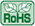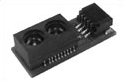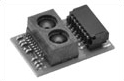# Products Lineup

## Distance Measuring Sensors <CMOS type>Analog Output (including I2C output) (Ta = 25°C)
Model No.
Distance
measuring
range
(cm)
Features
Absolute
maximum
ratings
Electro-optical characteristics*1
Vcc
(V)
Topr
(°C)
VOH
(V)
MIN.
VOL
(V)
MAX.
Dissipation
current
Operating
(mA)
4 to 50
Infrared LED and CMOS image sensor with built-in signal processing circuit,
compact size (18.9 × 8 × 5.2 mm),
high-precision measurement, analog output
–0.3 to +3.6
–10 to +60
VOUT(A)1=0.3 to 0.8 V
(at L = 50 cm),
VOUT(A)3=2.1 to 2.3 V
(at L = 4 cm)
MAX. 36
4 to 50
Infrared LED and CMOS image sensor with built-in signal processing circuit,
compact size (18.9 × 8 × 5.2 mm),
high-precision measurement, I2C output
–0.3 to +3.6
–10 to +60
D1 = 45 to 55 cm
(at L = 50 cm),
D3 = 3 to 5 cm
(at L = 4 cm)
MAX. 36
4 to 50
Infrared LED and CMOS image sensor with built-in signal processing circuit,
compact size (16.7 × 11 × 5.2 mm),
high-precision measurement,
analog / I2C output both compatible
–0.3 to +5.5
–10 to +60
VOUT(A)1=0.3 to 0.8 V,
D1 = 45 to 55 cm
(at L = 50 cm),
VOUT(A)3=2.1 to 2.3 V,
D3 = 3 to 5 cm
(at L = 4 cm)
MAX. 36
*1 Vcc = 3.3 VGP2Y0E02A(GP2Y0E02B) GP2Y0E03